# Face Generation¶

This project will use generative adversarial networks to generate new images of faces.

### Get the Data¶

Two datasets will be used in this project:

• MNIST
• CelebA
In :
data_dir = './data'

# FloydHub - Use with data ID "R5KrjnANiKVhLWAkpXhNBe"
#data_dir = '/input'

"""
DON'T MODIFY ANYTHING IN THIS CELL
"""
import helper


Found mnist Data
Found celeba Data


## Explore the Data¶

### MNIST¶

As you're aware, the MNIST dataset contains images of handwritten digits. You can view the first number of examples by changing show_n_images.

In :
show_n_images = 25

"""
DON'T MODIFY ANYTHING IN THIS CELL
"""
%matplotlib inline
import os
from glob import glob
from matplotlib import pyplot

mnist_images = helper.get_batch(glob(os.path.join(data_dir, 'mnist/*.jpg'))[:show_n_images], 28, 28, 'L')
pyplot.imshow(helper.images_square_grid(mnist_images, 'L'), cmap='gray')

Out:
<matplotlib.image.AxesImage at 0x7f100b5cd4e0>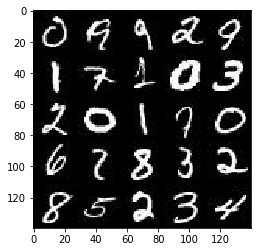### CelebA¶

The CelebFaces Attributes Dataset (CelebA) dataset contains over 200,000 celebrity images with annotations. Since you're going to be generating faces, you won't need the annotations. You can view the first number of examples by changing show_n_images.

In :
show_n_images = 25

"""
DON'T MODIFY ANYTHING IN THIS CELL
"""
mnist_images = helper.get_batch(glob(os.path.join(data_dir, 'img_align_celeba/*.jpg'))[:show_n_images], 40, 40, 'RGB')
pyplot.imshow(helper.images_square_grid(mnist_images, 'RGB'))

Out:
<matplotlib.image.AxesImage at 0x7f100b576da0>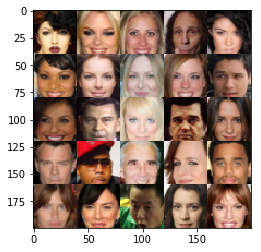## Preprocess the Data¶

Since the project's main focus is on building the GANs, we'll preprocess the data for you. The values of the MNIST and CelebA dataset will be in the range of -0.5 to 0.5 of 28x28 dimensional images. The CelebA images will be cropped to remove parts of the image that don't include a face, then resized down to 28x28.

The MNIST images are black and white images with a single color channel while the CelebA images have 3 color channels (RGB color channel).

## Build the Neural Network¶

You'll build the components necessary to build a GANs by implementing the following functions below:

• model_inputs
• discriminator
• generator
• model_loss
• model_opt
• train

This will check to make sure you have the correct version of TensorFlow and access to a GPU

In :
"""
DON'T MODIFY ANYTHING IN THIS CELL
"""
from distutils.version import LooseVersion
import warnings
import tensorflow as tf

# Check TensorFlow Version
assert LooseVersion(tf.__version__) >= LooseVersion('1.0'), 'Please use TensorFlow version 1.0 or newer.  You are using {}'.format(tf.__version__)
print('TensorFlow Version: {}'.format(tf.__version__))

# Check for a GPU
if not tf.test.gpu_device_name():
warnings.warn('No GPU found. Please use a GPU to train your neural network.')
else:
print('Default GPU Device: {}'.format(tf.test.gpu_device_name()))

TensorFlow Version: 1.0.1
Default GPU Device: /gpu:0


### Input¶

Implement the model_inputs function to create TF Placeholders for the Neural Network. It should create the following placeholders:

• Real input images placeholder with rank 4 using image_width, image_height, and image_channels.
• Z input placeholder with rank 2 using z_dim.
• Learning rate placeholder with rank 0.

Return the placeholders in the following the tuple (tensor of real input images, tensor of z data)
Sai: Rank means number of valuables

In :
import problem_unittests as tests

def model_inputs(image_width, image_height, image_channels, z_dim):
"""
Create the model inputs
:param image_width: The input image width
:param image_height: The input image height
:param image_channels: The number of image channels
:param z_dim: The dimension of Z
:return: Tuple of (tensor of real input images, tensor of z data, learning rate)
"""
# TODO: Implement Function

# For discriminator
inputs_real = tf.placeholder(tf.float32, (None, image_width, image_height, image_channels), name='input_real')
# For generator
inputs_z = tf.placeholder(tf.float32, (None, z_dim), name='input_z')

input_lr = tf.placeholder(tf.float32, name='input_lr')

return inputs_real, inputs_z, input_lr

"""
DON'T MODIFY ANYTHING IN THIS CELL THAT IS BELOW THIS LINE
"""
tests.test_model_inputs(model_inputs)

Tests Passed


### Discriminator¶

Implement discriminator to create a discriminator neural network that discriminates on images. This function should be able to reuse the variabes in the neural network. Use tf.variable_scope with a scope name of "discriminator" to allow the variables to be reused. The function should return a tuple of (tensor output of the discriminator, tensor logits of the discriminator).

In :
def discriminator(images, reuse=False):
"""
Create the discriminator network
:param image: Tensor of input image(s)
:param reuse: Boolean if the weights should be reused
:return: Tuple of (tensor output of the discriminator, tensor logits of the discriminator)
"""
# TODO: Implement Function
alpha=0.2 # Alpha for Leaky ReLU
# print(images)
with tf.variable_scope('discriminator', reuse=reuse):
# Input layer is 28x28x3, Kernel size 5x5
x1 = tf.layers.conv2d(images, 64, 5, strides=2, padding='same')
relu1 = tf.maximum(alpha * x1, x1) # leaky ReLU.
# 14x14x64

x2 = tf.layers.conv2d(relu1, 128, 5, strides=2, padding='same')
bn2 = tf.layers.batch_normalization(x2, training=True)
relu2 = tf.maximum(alpha * bn2, bn2)
# 7x7x128

x3 = tf.layers.conv2d(relu2, 256, 5, strides=2, padding='same')
bn3 = tf.layers.batch_normalization(x3, training=True)
relu3 = tf.maximum(alpha * bn3, bn3) # N units in this layer.
# 4x4x256

# Flatten it, 姐係Reshape it into one long vector
flat = tf.reshape(relu3, (-1, 4*4*256)) #  4096 units in this layer.
logits = tf.layers.dense(flat, 1) # fully connected layer，【 Only one unit】
out = tf.sigmoid(logits) # sigmoid cross entropy loss calculation

return out, logits

"""
DON'T MODIFY ANYTHING IN THIS CELL THAT IS BELOW THIS LINE
"""
tests.test_discriminator(discriminator, tf)

Tests Passed


### Generator¶

Implement generator to generate an image using z. This function should be able to reuse the variabes in the neural network. Use tf.variable_scope with a scope name of "generator" to allow the variables to be reused. The function should return the generated 28 x 28 x out_channel_dim images.

In :
# Sai: This time, it's assumed that reuse=False for training and reuse=True for inference
def generator(z, out_channel_dim, is_train=True):
"""
Create the generator network
:param z: Input z
:param out_channel_dim: The number of channels in the output image
:param is_train: Boolean if generator is being used for training
:return: The tensor output of the generator
"""
# TODO: Implement Function
alpha=0.2 # Alpha for Leaky ReLU
# print(z)
with tf.variable_scope('generator', reuse=(not is_train)):
# First fully connected layer
x1 = tf.layers.dense(z, 2*2*512) #第一件平面野。
# Reshape it to start the convolutional stack
x1 = tf.reshape(x1, (-1, 2, 2, 512)) #第二件立體長條野。
# x1 = tf.layers.batch_normalization(x1, training=is_train) #Batch normalization
x1 = tf.maximum(alpha * x1, x1) # Leaky ReLU
# 2x2x512 now

x2 = tf.layers.conv2d_transpose(x1, 256, 5, strides=2, padding='valid')
x2 = tf.layers.batch_normalization(x2, training=is_train)
x2 = tf.maximum(alpha * x2, x2)
# 7x7x256 now

x3 = tf.layers.conv2d_transpose(x2, 28, 5, strides=2, padding='same')
x3 = tf.layers.batch_normalization(x3, training=is_train)
x3 = tf.maximum(alpha * x3, x3)
# 14x14x28 now

# Output layer
logits = tf.layers.conv2d_transpose(x3, out_channel_dim, 5, strides=2, padding='same')
# 28x28xOut_channel_dim now

out = tf.tanh(logits)
return out

"""
DON'T MODIFY ANYTHING IN THIS CELL THAT IS BELOW THIS LINE
"""
tests.test_generator(generator, tf)

Tests Passed


### Loss¶

Implement model_loss to build the GANs for training and calculate the loss. The function should return a tuple of (discriminator loss, generator loss). Use the following functions you implemented:

• discriminator(images, reuse=False)
• generator(z, out_channel_dim, is_train=True)
In :
def model_loss(input_real, input_z, out_channel_dim):
"""
Get the loss for the discriminator and generator
:param input_real: Images from the real dataset
:param input_z: Z input
:param out_channel_dim: The number of channels in the output image
:return: A tuple of (discriminator loss, generator loss)

zeros_like: Creates a tensor with all elements set to 0.
ones_like: Creates a tensor with all elements set to 1.
"""
# TODO: Implement Function

g_model = generator(input_z, out_channel_dim)
d_model_real, d_logits_real = discriminator(input_real)
d_model_fake, d_logits_fake = discriminator(g_model, reuse=True)

d_loss_real = tf.reduce_mean(
tf.nn.sigmoid_cross_entropy_with_logits(logits=d_logits_real, labels=tf.ones_like(d_model_real)))
d_loss_fake = tf.reduce_mean(
tf.nn.sigmoid_cross_entropy_with_logits(logits=d_logits_fake, labels=tf.zeros_like(d_model_fake)))
g_loss = tf.reduce_mean(
tf.nn.sigmoid_cross_entropy_with_logits(logits=d_logits_fake, labels=tf.ones_like(d_model_fake)))

d_loss = d_loss_real + d_loss_fake

return d_loss, g_loss

"""
DON'T MODIFY ANYTHING IN THIS CELL THAT IS BELOW THIS LINE
"""
tests.test_model_loss(model_loss)

Tests Passed


### Optimization¶

Implement model_opt to create the optimization operations for the GANs. Use tf.trainable_variables to get all the trainable variables. Filter the variables with names that are in the discriminator and generator scope names. The function should return a tuple of (discriminator training operation, generator training operation).

In :
def model_opt(d_loss, g_loss, learning_rate, beta1):
"""
Get optimization operations
:param d_loss: Discriminator loss Tensor
:param g_loss: Generator loss Tensor
:param learning_rate: Learning Rate Placeholder
:param beta1: The exponential decay rate for the 1st moment in the optimizer
:return: A tuple of (discriminator training operation, generator training operation)
"""
# TODO: Implement Function

# Get weights and bias to update
t_vars = tf.trainable_variables()
d_vars = [var for var in t_vars if var.name.startswith('discriminator')]
g_vars = [var for var in t_vars if var.name.startswith('generator')]
""""
print("===============Start Optimizers===============")
print("Variable of discriminator:")
for d_var in d_vars:
print(d_var.name)
print("\nVariable of generator:")
for g_var in g_vars:
print(g_var.name)
print("===============End Optimizers===============")
"""

# Optimize
with tf.control_dependencies(tf.get_collection(tf.GraphKeys.UPDATE_OPS)):

return d_train_opt, g_train_opt

"""
DON'T MODIFY ANYTHING IN THIS CELL THAT IS BELOW THIS LINE
"""
tests.test_model_opt(model_opt, tf)

Tests Passed


## Neural Network Training¶

### Show Output¶

Use this function to show the current output of the generator during training. It will help you determine how well the GANs is training.

In :
"""
DON'T MODIFY ANYTHING IN THIS CELL
"""
import numpy as np

def show_generator_output(sess, n_images, input_z, out_channel_dim, image_mode):
"""
Show example output for the generator
:param sess: TensorFlow session
:param n_images: Number of Images to display
:param input_z: Input Z Tensor
:param out_channel_dim: The number of channels in the output image
:param image_mode: The mode to use for images ("RGB" or "L")
"""
cmap = None if image_mode == 'RGB' else 'gray'
z_dim = input_z.get_shape().as_list()[-1]
example_z = np.random.uniform(-1, 1, size=[n_images, z_dim])

samples = sess.run(
generator(input_z, out_channel_dim, False),
feed_dict={input_z: example_z})

images_grid = helper.images_square_grid(samples, image_mode)
pyplot.imshow(images_grid, cmap=cmap)
pyplot.show()


### Train¶

Implement train to build and train the GANs. Use the following functions you implemented:

• model_inputs(image_width, image_height, image_channels, z_dim)
• model_loss(input_real, input_z, out_channel_dim)
• model_opt(d_loss, g_loss, learning_rate, beta1)

Use the show_generator_output to show generator output while you train. Running show_generator_output for every batch will drastically increase training time and increase the size of the notebook. It's recommended to print the generator output every 100 batches.

In :
def train(epoch_count, batch_size, z_dim, learning_rate, beta1, get_batches, data_shape, data_image_mode):
"""
Train the GAN
:param epoch_count: Number of epochs
:param batch_size: Batch Size
:param z_dim: Z dimension
:param learning_rate: Learning Rate
:param beta1: The exponential decay rate for the 1st moment in the optimizer
:param get_batches: Function to get batches
:param data_shape: Shape of the data
:param data_image_mode: The image mode to use for images ("RGB" or "L")
"""
# TODO: Build Model

#====================Init Part Start=============================
steps = 0
show_every = 100 #Recommended to print the generator output every 100 batches
print_every = 10

#(image_width, image_height, image_channels, z_dim)

n_samples, width, height, channels = data_shape
input_real, input_z, input_lr = model_inputs(width, height, channels, z_dim)
d_loss, g_loss = model_loss(input_real, input_z, channels)
d_opt, g_opt = model_opt(d_loss, g_loss, input_lr, beta1)
#====================Init Part End=============================

with tf.Session() as sess:
# saver = tf.train.Saver()
sess.run(tf.global_variables_initializer())
for epoch_i in range(epoch_count):
for batch_images in get_batches(batch_size):

# TODO: Train Model
steps += 1

# Sample random noise for G
batch_z = np.random.uniform(-1, 1, size=(batch_size, z_dim))

# Run optimizers
_ = sess.run(d_opt, feed_dict={input_real: batch_images, input_z: batch_z, input_lr: learning_rate})
_ = sess.run(g_opt, feed_dict={input_real: batch_images, input_z: batch_z, input_lr: learning_rate})

if steps % print_every == 0:
# At the end of each epoch, get the losses and print them out
train_loss_d = d_loss.eval({input_z: batch_z, input_real: batch_images})
train_loss_g = g_loss.eval({input_z: batch_z})

print("Epoch {}/{}...".format(epoch_i, epoch_count),
"Discriminator Loss: {:.4f}...".format(train_loss_d),
"Generator Loss: {:.4f}".format(train_loss_g))
# Save losses to view after training
# losses.append((train_loss_d, train_loss_g))

if steps % show_every == 0:
n_images = 25
show_generator_output(sess, n_images, input_z, channels, data_image_mode)

#    saver.save(sess, './checkpoints/generator.ckpt')

#with open('samples.pkl', 'wb') as f:
#    pkl.dump(samples, f)



### MNIST¶

Test your GANs architecture on MNIST. After 2 epochs, the GANs should be able to generate images that look like handwritten digits. Make sure the loss of the generator is lower than the loss of the discriminator or close to 0.

In :
batch_size = 64
z_dim = 50
learning_rate = 0.0002
beta1 = 0.5 # For Adam Optimizer

"""
DON'T MODIFY ANYTHING IN THIS CELL THAT IS BELOW THIS LINE
"""
epochs = 2

mnist_dataset = helper.Dataset('mnist', glob(os.path.join(data_dir, 'mnist/*.jpg')))
with tf.Graph().as_default():
train(epochs, batch_size, z_dim, learning_rate, beta1, mnist_dataset.get_batches,
mnist_dataset.shape, mnist_dataset.image_mode)

Epoch 0/2... Discriminator Loss: 0.3529... Generator Loss: 1.7011
Epoch 0/2... Discriminator Loss: 1.6074... Generator Loss: 0.5936
Epoch 0/2... Discriminator Loss: 0.9963... Generator Loss: 0.9465
Epoch 0/2... Discriminator Loss: 1.0408... Generator Loss: 1.0673
Epoch 0/2... Discriminator Loss: 1.2708... Generator Loss: 0.4532
Epoch 0/2... Discriminator Loss: 0.7908... Generator Loss: 1.1342
Epoch 0/2... Discriminator Loss: 0.8518... Generator Loss: 0.9085
Epoch 0/2... Discriminator Loss: 0.8862... Generator Loss: 0.7493
Epoch 0/2... Discriminator Loss: 0.5988... Generator Loss: 1.6510
Epoch 0/2... Discriminator Loss: 0.9425... Generator Loss: 3.2277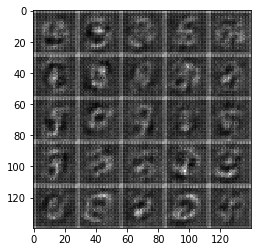Epoch 0/2... Discriminator Loss: 0.9785... Generator Loss: 0.5607
Epoch 0/2... Discriminator Loss: 0.4214... Generator Loss: 2.6535
Epoch 0/2... Discriminator Loss: 1.3136... Generator Loss: 0.3609
Epoch 0/2... Discriminator Loss: 0.2593... Generator Loss: 2.8853
Epoch 0/2... Discriminator Loss: 0.4901... Generator Loss: 1.1977
Epoch 0/2... Discriminator Loss: 2.0580... Generator Loss: 0.1699
Epoch 0/2... Discriminator Loss: 0.5793... Generator Loss: 5.5373
Epoch 0/2... Discriminator Loss: 0.4568... Generator Loss: 1.2137
Epoch 0/2... Discriminator Loss: 1.3392... Generator Loss: 0.4131
Epoch 0/2... Discriminator Loss: 0.1797... Generator Loss: 2.7855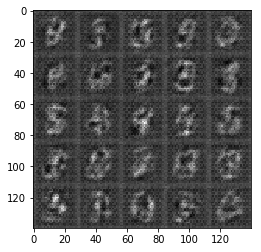Epoch 0/2... Discriminator Loss: 1.3596... Generator Loss: 8.2312
Epoch 0/2... Discriminator Loss: 0.8998... Generator Loss: 0.7192
Epoch 0/2... Discriminator Loss: 0.5116... Generator Loss: 1.3081
Epoch 0/2... Discriminator Loss: 0.2689... Generator Loss: 3.2931
Epoch 0/2... Discriminator Loss: 0.3671... Generator Loss: 3.1152
Epoch 0/2... Discriminator Loss: 0.4063... Generator Loss: 1.7685
Epoch 0/2... Discriminator Loss: 0.2530... Generator Loss: 2.0219
Epoch 0/2... Discriminator Loss: 0.3977... Generator Loss: 1.4248
Epoch 0/2... Discriminator Loss: 0.2442... Generator Loss: 2.0028
Epoch 0/2... Discriminator Loss: 0.1850... Generator Loss: 4.3407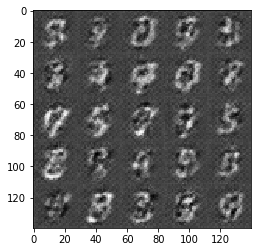Epoch 0/2... Discriminator Loss: 0.1158... Generator Loss: 2.9981
Epoch 0/2... Discriminator Loss: 0.2360... Generator Loss: 2.6501
Epoch 0/2... Discriminator Loss: 0.2709... Generator Loss: 1.8519
Epoch 0/2... Discriminator Loss: 0.2189... Generator Loss: 3.0186
Epoch 0/2... Discriminator Loss: 0.0800... Generator Loss: 3.4814
Epoch 0/2... Discriminator Loss: 0.1351... Generator Loss: 2.6641
Epoch 0/2... Discriminator Loss: 0.1785... Generator Loss: 2.2863
Epoch 0/2... Discriminator Loss: 0.1737... Generator Loss: 2.2589
Epoch 0/2... Discriminator Loss: 0.9332... Generator Loss: 0.6086
Epoch 0/2... Discriminator Loss: 0.4850... Generator Loss: 1.3295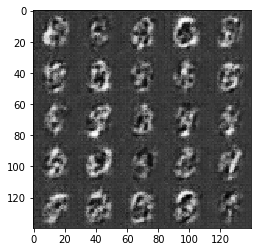Epoch 0/2... Discriminator Loss: 0.3619... Generator Loss: 1.7675
Epoch 0/2... Discriminator Loss: 0.2466... Generator Loss: 2.0858
Epoch 0/2... Discriminator Loss: 0.2285... Generator Loss: 2.0869
Epoch 0/2... Discriminator Loss: 0.2463... Generator Loss: 1.9138
Epoch 0/2... Discriminator Loss: 0.1112... Generator Loss: 3.2264
Epoch 0/2... Discriminator Loss: 0.1469... Generator Loss: 2.4068
Epoch 0/2... Discriminator Loss: 0.0881... Generator Loss: 2.9653
Epoch 0/2... Discriminator Loss: 0.0999... Generator Loss: 2.9049
Epoch 0/2... Discriminator Loss: 0.0644... Generator Loss: 3.7905
Epoch 0/2... Discriminator Loss: 0.1150... Generator Loss: 2.8227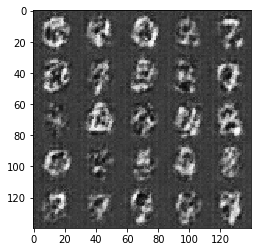Epoch 0/2... Discriminator Loss: 0.0820... Generator Loss: 3.6289
Epoch 0/2... Discriminator Loss: 0.0627... Generator Loss: 3.4280
Epoch 0/2... Discriminator Loss: 0.0655... Generator Loss: 3.4264
Epoch 0/2... Discriminator Loss: 0.0474... Generator Loss: 4.0668
Epoch 0/2... Discriminator Loss: 0.0671... Generator Loss: 3.5017
Epoch 0/2... Discriminator Loss: 0.0394... Generator Loss: 4.2797
Epoch 0/2... Discriminator Loss: 0.0506... Generator Loss: 4.1242
Epoch 0/2... Discriminator Loss: 0.0733... Generator Loss: 3.0829
Epoch 0/2... Discriminator Loss: 0.8633... Generator Loss: 1.7021
Epoch 0/2... Discriminator Loss: 0.6786... Generator Loss: 1.0644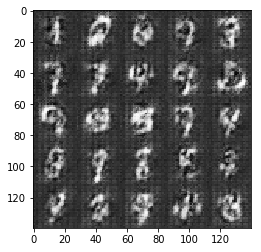Epoch 0/2... Discriminator Loss: 0.4827... Generator Loss: 1.7022
Epoch 0/2... Discriminator Loss: 0.3208... Generator Loss: 1.6744
Epoch 0/2... Discriminator Loss: 0.2469... Generator Loss: 2.0421
Epoch 0/2... Discriminator Loss: 0.2864... Generator Loss: 1.7591
Epoch 0/2... Discriminator Loss: 0.2195... Generator Loss: 2.3634
Epoch 0/2... Discriminator Loss: 0.2446... Generator Loss: 2.0050
Epoch 0/2... Discriminator Loss: 0.1635... Generator Loss: 2.5166
Epoch 0/2... Discriminator Loss: 0.3119... Generator Loss: 1.7848
Epoch 0/2... Discriminator Loss: 0.2239... Generator Loss: 2.1831
Epoch 0/2... Discriminator Loss: 0.1467... Generator Loss: 2.5093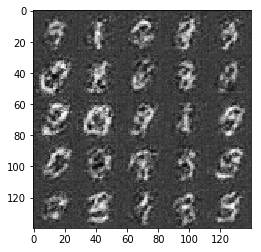Epoch 0/2... Discriminator Loss: 0.2340... Generator Loss: 1.9044
Epoch 0/2... Discriminator Loss: 0.3629... Generator Loss: 1.4153
Epoch 0/2... Discriminator Loss: 0.2821... Generator Loss: 1.6817
Epoch 0/2... Discriminator Loss: 0.1590... Generator Loss: 2.5161
Epoch 0/2... Discriminator Loss: 0.1523... Generator Loss: 2.4117
Epoch 0/2... Discriminator Loss: 0.2054... Generator Loss: 2.1265
Epoch 0/2... Discriminator Loss: 0.1729... Generator Loss: 2.3309
Epoch 0/2... Discriminator Loss: 0.2218... Generator Loss: 2.0150
Epoch 0/2... Discriminator Loss: 0.1539... Generator Loss: 2.3611
Epoch 0/2... Discriminator Loss: 0.1401... Generator Loss: 2.6046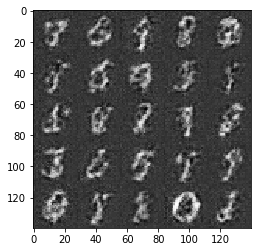Epoch 0/2... Discriminator Loss: 0.1218... Generator Loss: 2.6944
Epoch 0/2... Discriminator Loss: 0.1230... Generator Loss: 2.7044
Epoch 0/2... Discriminator Loss: 0.1208... Generator Loss: 2.6811
Epoch 0/2... Discriminator Loss: 0.1699... Generator Loss: 2.3374
Epoch 0/2... Discriminator Loss: 0.1140... Generator Loss: 2.7898
Epoch 0/2... Discriminator Loss: 0.0955... Generator Loss: 2.8189
Epoch 0/2... Discriminator Loss: 0.1359... Generator Loss: 2.8461
Epoch 0/2... Discriminator Loss: 0.1280... Generator Loss: 2.6488
Epoch 0/2... Discriminator Loss: 0.1115... Generator Loss: 2.9215
Epoch 0/2... Discriminator Loss: 0.0836... Generator Loss: 3.1238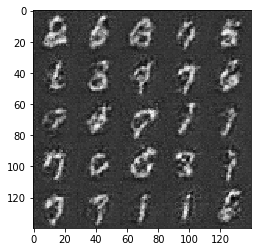Epoch 0/2... Discriminator Loss: 0.1268... Generator Loss: 2.6280
Epoch 0/2... Discriminator Loss: 0.0569... Generator Loss: 3.8456
Epoch 0/2... Discriminator Loss: 0.1748... Generator Loss: 2.4415
Epoch 1/2... Discriminator Loss: 0.0602... Generator Loss: 3.9264
Epoch 1/2... Discriminator Loss: 0.1872... Generator Loss: 2.1417
Epoch 1/2... Discriminator Loss: 0.1088... Generator Loss: 3.0850
Epoch 1/2... Discriminator Loss: 0.1940... Generator Loss: 2.0609
Epoch 1/2... Discriminator Loss: 0.0829... Generator Loss: 3.2476
Epoch 1/2... Discriminator Loss: 15.4432... Generator Loss: 14.9406
Epoch 1/2... Discriminator Loss: 0.8730... Generator Loss: 1.0388